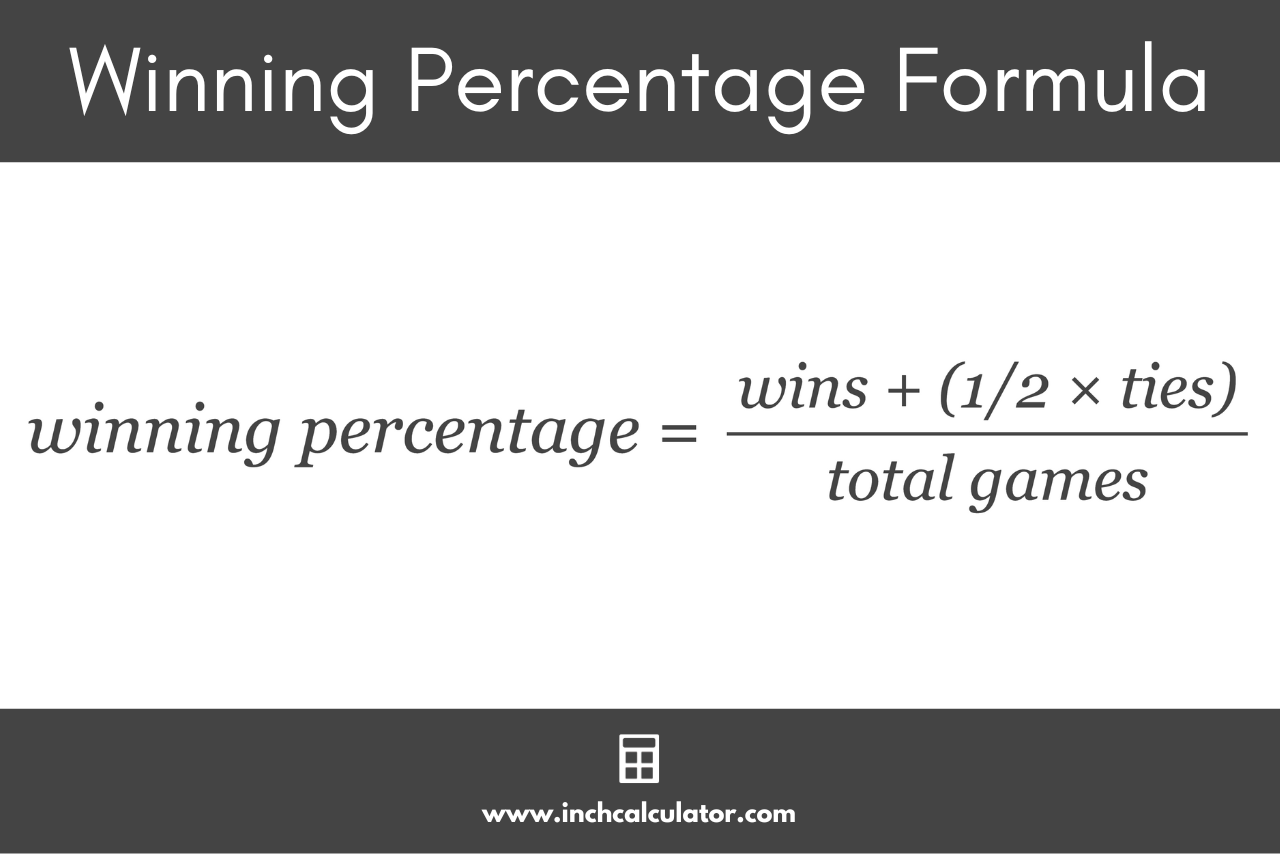# Winning Percentage Calculator

Use our winning percentage calculator to find the percentage of wins given the total wins, losses, and ties.

## Winning Percentage:

### Steps to Solve Using the Win Percentage Formula

win pct = wins + (1/2 × ties) / total games

Learn how we calculated this below

## How to Calculate the Winning Percentage

A team’s winning percentage is a fundamental statistic used in various competitive arenas, particularly in sports. At its core, a winning percentage represents the percentage of games or matches a team or individual has won relative to the total number they have participated in.

You can calculate a winning percentage using a mathematical formula.

### Winning Percentage Formula

The formula for calculating the winning percentage is:

winning percentage = wins + (½ × ties) / total games

The winning percentage is equal to the number of wins plus one-half of the ties, divided by the total number of games played. The winning percentage can be expressed as a ratio of the wins to the total number of games played.If the result is a decimal, you can round to the nearest whole number or keep it as a decimal, depending on your preference and the level of precision you desire.

Unlike a probability, the winning percentage is the ratio of wins to total games that have actually taken place, rather than the likelihood of winning the next game played.

For example, suppose a basketball team has played 50 games in a season. Out of these, they’ve won 30 games. Let’s calculate their winning percentage using the formula above.

winning percentage = 30 / 50 = 60%

This means the basketball team has a winning percentage of 60% for the season.